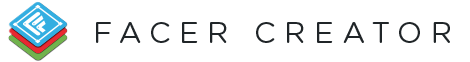#Math expressions can take your watch face to next level. Facer supports arithmetical operations as well as a handful of math functions. You can also drop facer tags into expressions to make them even more dynamic!

NOTE: Math expression require parentheses. So, this will work `(1+1)` and this will not `1+1` !

## Operators

OPERATOR DEFINITION EXAMPLE EXPRESSION EXAMPLE OUTPUT
- Subtraction operator (3-2) 1
* Multiplication operator (3*2) 6
/ Division operator (3/2) 1.5
% Modulo operator (5%2) 1

## Constants

COSTANT DEFINITION EXAMPLE EXPRESSION EXAMPLE OUTPUT
pi Value of pi (pi) 3.141592653589793
e Value of e (e) 2.718281828459045

## Functions

FUNCTION DEFINITION EXAMPLE EXPRESSION EXAMPLE OUTPUT
rand(min, max) Random number generator (rand(1, 10)) 1
wakeRand(min, max) Random number generator that's updated every time the watch face goes into active mode (wakeRand(1, 10)) 1
abs(number) Absolute value function (abs(-10)) 10
sin(number) Sine function (sin(1)) 0.8414709848078965
cos(number) Cosine function (cos(1)) 0.5403023058681398
tan(number) Tangent function (tan(1)) 1.5574077246549023
round(number) Round function (get the closest integer) (round(1.6)) 2
ceil(number) Ceiling function (gets the next highest integer) (ceil(1.2)) 2
floor(number) Floor function - gets the next lowest integer) (floor(1.6)) 1
log(number) Log function (log(2)) 0.6931471805599453
log2(number) Log base 2 function (log2(2)) 1
log10(number) Log base 10 function (log10(2)) 0.3010299956639812
sqrt(number) Square root function (sqrt(4)) 2
cbrt(number) Cube root function (cbrt(8)) 2
exp(number) Gets E to the x power (exp(2)) 7.38905609893065
expm1(number) Gets E to the x power minus 1 (expm1(2)) 6.38905609893065